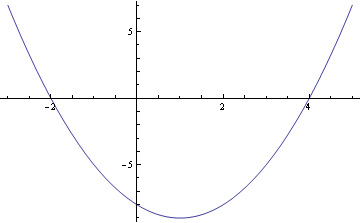# Without Solving The Equation (or Factoring), Can You Determine The Solutions To The Equation X²-2x-8 = 0 By Inspecting The Graph? Does It Have A Maximum Or A Minimum

## 1 Answers

Oddman answered
In order to determine the solution from a graph, you need to have a graph. The picture is a graph of the function f[x] = x2 -2x -8.

The solutions to f[x]=0 are given by the x-intercepts of the graph of f[x]. These are x=-2, and x=4.
The graph has a minimum at (x, y) = (1, -9).thanked the writer.

## Answer Question